Courses
Courses for Kids
Free study material
Offline Centres
More

# Thermal Properties of Matter Class 11 Notes CBSE Physics Chapter 11 (Free PDF Download)Last updated date: 06th Dec 2023
Total views: 654.3k
Views today: 12.54k

## Revision Notes for CBSE Class 11 Physics Chapter 11 (Thermal Properties of Matter) - Free PDF Download

CBSE prescribes the NCERT textbook for strengthening the base of the students studying in Class 11 PCM. It is evident that studying in the CBSE board increases the probability of students achieving high scores in AIEEE, IIT-JEE, NEET, and AIPMT. From Chapter 11 Thermal Properties of Matter, you can expect one question with a weightage of 2% in the NEET exam and three questions from the topic of Heat & Thermodynamics in the JEE Main exam.

Also, check CBSE Class 11 Physics revision notes for other chapters:

Thermal Properties of Matter Chapter-Related Important Study Materials
It is a curated compilation of relevant online resources that complement and expand upon the content covered in a specific chapter. Explore these links to access additional readings, explanatory videos, practice exercises, and other valuable materials that enhance your understanding of the chapter's subject matter.

 Thermal Properties of Matter Related Other Study Materials Thermal Properties of Matter Important Questions Thermal Properties of Matter NCERT Solutions Thermal Properties of Matter NCERT Exemplar Thermal Properties of Matter NCERT Formulas

## Thermal Properties of Matter Class 11 Notes Physics - Basic Subjective Questions

### Section – A (1 Mark Questions)

1. Three bodies are having temperature $T_{A}=-42^{\circ}F,T_{B}=-10^{\circ}C,T_{C}=200K$ . Which body among these is most warm?

Ans. Let us calculate all body temperature on same scale

Let choose Celsius

C=K-273.15

C=(5F-160)/9

$T_{A}=-42^{\circ}F=(5\times -42-160)/9=-41^{\circ}C$

$T_{B}=-10^{\circ}C$

$T_{C}=200K=200-273\cdot 15=-73\cdot 1^{\circ}C$

So the most warm body is TB.

2. If a thermometer reads freezing point of water as 20°C and boiling point as 150°C, how much does the thermometer read, when the actual temperature is 60°C?

Ans. 150C-20C=100x

1x=1.3C

thermometer reading = 1.3C + 20

$=1\cdot 3(60)+20=98^{\circ}C$

3. Burns from steam are usually more serious than those from boiling water. Why ?

Ans. Steam will produce more severe burns than boiling water because steam has more heat energy than water due to its latent heat of vaporization.

For water, the latent heat of fusion and vaporization are, $L_{f}=3\cdot 33\times 10^{5}Jkg^{-1}$ and $L_{V}=22\cdot 6\times 10^{5}Jkg^{-1}$ respectively. It means $22\cdot 6\times 10^{5}Jkg^{-1}$ of heat is needed to convert 1 kg of water to steam at 100°C. So, steam at 100°C carries more heat than water at 100°C. This is why burns from steam are usually more serious than those from boiling water.

4. A bimetallic strip consists of brass and iron when it is heated it bends into an arc with brass on the convex and iron on the concave side of the arc. Why does this happen?

Ans. Two strips of equal lengths but of different materials (different coefficient of linear expansion $\varpropto$ ) when joined together, is called a bimetallic strip. This strip has the characteristic property of bending on heating due to unequal linear expansion of the two metals. The brass side bends on outer side (convex side) due to greater $\varpropto$ and iron bends on inner side (concave side) due to smaller $\varpropto$ .

5. Why are birds often seen to swell their feathers in winter?

Ans. When birds swell their feathers, they trap air in the feather. Air being a poor conductor prevents loss of heat and keeps the bird warm.

### Section – B (2 Marks Questions)

6. The reading of the Centigrade thermometer coincides with that of the Fahrenheit thermometer in a liquid. Then find the temperature of the liquid.

Ans. $\dfrac{C}{5}=\dfrac{F-32}{9}4$

C=F=x

$\dfrac{x}{5}=\dfrac{x-32}{9}$

$9x=5x-32\times 5$

$4x=-32\times 5$

$x=\dfrac{-32\times 5}{4}=-40^{\circ}C$

7. Two metal rods A and B are having their initial lengths in the ratio 2 : 3 and the coefficient of linear expansion in the ratio 3 : 4. When they are heated through the same temperature difference, then find the ratio of their change in length or expansion.

Ans. $\dfrac{lA}{lB}=\dfrac{2}{3};\dfrac{\varpropto_{A}}{\varpropto_{B}}=\dfrac{3}{4}$

$\dfrac{\Delta lA}{\Delta lB}=\dfrac{l_{A}\varpropto_{A}\Delta T}{l_{b}\varpropto_{B}\Delta T}=\left ( \dfrac{l_{A}}{l_{B}} \right )\left ( \dfrac{\varpropto_{A}}{\varpropto_{B}} \right )=\dfrac{2}{3}\times \dfrac{3}{4}=1:2$

8. The surface temperature of the sun which has maximum energy emission at 500 nm is 6000 K. Now find the temperature of a star which has maximum energy emission at 400 nm.

Ans. By using Wien’s displacement law,

$(\lambda _{m})_{1}T_{1}=(\lambda _{m})_{2}T_{2}$

$\therefore 500\times 6000=400\times T_{2}$

$\Rightarrow T_{2}=\dfrac{500\times 6000}{400}$

=7500K

9. Ice starts forming in a lake with water at 0°C, when atmospheric temperature is –10°C. If the time taken for 5mm thickness of ice to be formed is 2h, then find the time taken for the thickness of ice to change from 5mm to 20 mm.

Ans. $t=\dfrac{\rho L}{2K\theta }\left ( x_{1}^{2}-x_{2}^{2} \right )\;t\varpropto\left ( x_{1}^{2}-x_{2}^{2} \right )$

$\dfrac{{t}'}{t}=\dfrac{(x'{_{1}^{2}}-x'{_{2}^{2}})}{(x_{1}^{2}-x_{2}^{2})}$

$\dfrac{{t}'}{2}=\dfrac{20^{2}-5^{2}}{5^{2}-0^{2}}$

${t}'=30h$

10. The volume of mercury in the bulb of a thermometer is 10-6 m3. The area of cross-section of the capillary tube is $2\times 10^{-7}m^{2}$ . If the temperature is raised by $100^{\circ}C$ , then find the increase in the length of the mercury column. $\left ( Take,\gamma _{Hg}=18\times 10^{-5}/^{\circ}C \right )$

Ans. By cubical expansion relation,

$\Delta V=V\times _{\gamma }\Delta T$

Where, $\gamma =$ coefficient cubical expansion,

$V=10^{-6}m^{3}$

= initial volume

$\gamma =18\times 10^{-5}/^{\circ}C$

$\Delta T=100^{\circ}C$

$\Delta V=10^{-6}\times 18\times 10^{-5}\times 10^{2}$

$=18\times 10^{-9}m^{3}$ .

Since, $\Delta V=A\times \Delta l$

$\therefore 18\times 10^{-9}=2\times 10^{-7}\times \Delta l$

or $9\times 10^{-2}=\Delta l$

or $\Delta l=9cm$ .

PDF Summary - Class 11 Physics Thermal Properties of Matter Notes (Chapter 11)

1. Thermal Properties of Matter:

This topic discusses various thermal phenomena and how a matter behaves when subjected to the flow of thermal energy. We are specifically concerned in

• Thermal Expansion.

• Heat and Calorimetry

• Transfer of Heat

### 1.1 Temperature and Heat

Temperature:

• Temperature is a relative measure of a body's hotness or coldness.

• SI Unit: Kelvin (K)

• Commonly used unit: $\text{ }\!\!{}^\circ\!\!\text{ C or }\!\!{}^\circ\!\!\text{ F}$

• Conversion: $\text{t}\left( \text{K} \right)\text{ = t}\left( \text{ }\!\!{}^\circ\!\!\text{ C} \right)\text{ + 273}\text{.15}$

Heat:

• Heat is a type of energy flow that occurs,

i. between two bodies or

ii. between a body and its surroundings as a result of a temperature difference.

• SI unit: Joule (J)

• Commonly used unit: Calorie (Cal)

• Conversion: $\text{1 cal = 4}\text{.186 J}$

• Heat is always transferred from a higher temperature system to a lower temperature system.

### 1.2 Measurement of Temperature:

Principle:

• Thermometric properties are observed as temperature changes and compared to certain reference situations.

• Generally, the reference situation is ice point or steam point.

## 1.2.1 Celsius and Fahrenheit Temperature Scale:

 In Celsius Scale In Fahrenheit Scale $\text{Ice point }\to \text{ 0 }\!\!{}^\circ\!\!\text{ C}$ $\text{Ice point }\to \text{ 32 }\!\!{}^\circ\!\!\text{ F}$ $\text{Stream point }\to \text{ 1000 }\!\!{}^\circ\!\!\text{ C}$ $\text{Stream point }\to \text{ 212 }\!\!{}^\circ\!\!\text{ F}$

It implies that 100 divisions in Celsius are equivalent to 180 divisions in Fahrenheit.

Hence, $\dfrac{{{\text{t}}_{\text{f}}}\text{ }-\text{ }32}{180}\text{ = }\dfrac{{{\text{t}}_{\text{c}}}}{100}$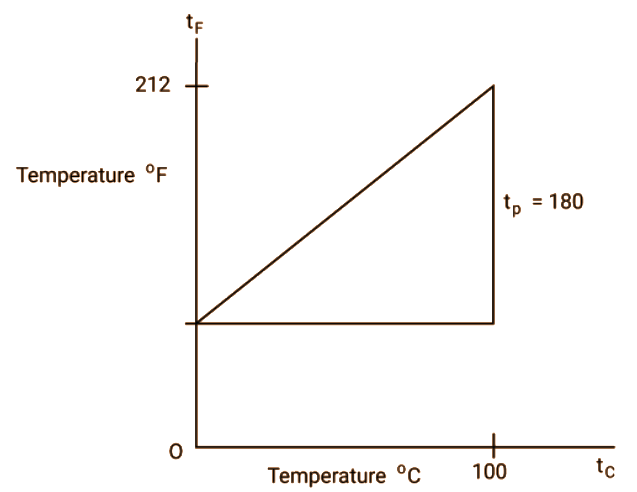### 1.2.2 Absolute Temperature Scale:

• It is kelvin scale

$\text{Ice point }\to \text{ 273}\text{.15 K}$

$\text{Steam point }\to \text{ 373}\text{.15 K}$

• When compared to the Celsius scale, the number of scale divisions in both scales is the same.

$\dfrac{{{\text{t}}_{\text{c}}}\text{ }-\text{ 0}{}^\circ \text{C}}{100}\text{ = }\dfrac{{{\text{t}}_{\text{k}}}-\text{ }273.15}{100}$

• The Kelvin scale is known as an absolute scale because it is nearly impossible to go beyond 0 K on the negative side.

(Image will be updated soon)

1.2.3 Thermometers:

• A thermometer is a device used to measure the temperature of any system. The liquid in glass thermometers, Platinum Resistance Thermometers, and Constant Volume Gas Thermometers are a few examples.

• The Liquid in Glass thermometer and the Platinum Resistance thermometer provide uniform readings for ice point and steam point but vary for different liquids and materials.

• A constant-volume gas thermometer provides the same readings regardless of which gas is used. It is based on the fact that at low pressures and constant volume, $\text{P }\!\!\times\!\!\text{ T}$ for a gas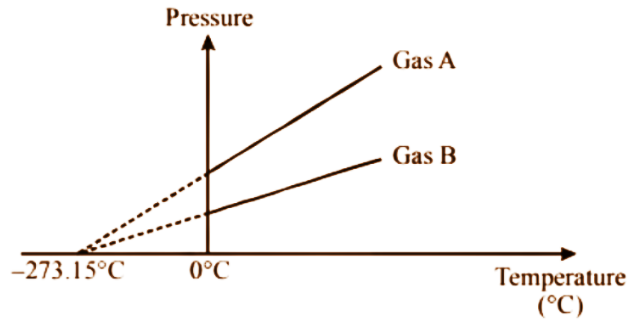• All gases collide to absolute zero at zero pressure.

 Type of expansion $\dfrac{\text{ }\!\!\Delta\!\!\text{ x}}{\text{x}}=\text{ K}\Delta \text{T}$ Constant (k) Linear Expansion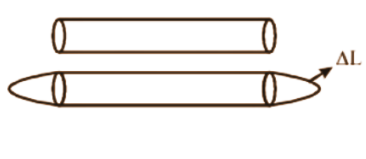$\dfrac{\text{ }\!\!\Delta\!\!\text{ L}}{\text{L}}=\text{ }\!\!\alpha\!\!\text{ }\Delta \text{T}$ Coefficient of linear expansion: It rises in length per unit length per degree increase in temperature. Area expansion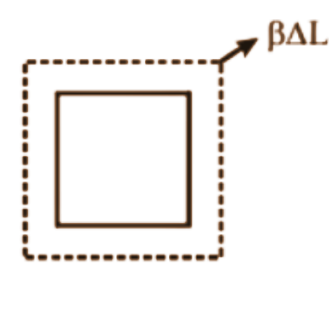$\dfrac{\text{ }\!\!\Delta\!\!\text{ A}}{\text{A}}=\text{ }\!\!\beta\!\!\text{ }\Delta \text{T}$ Coefficient of area expansion: It rises in area per unit area per degree increase in temperature. Volume Expansion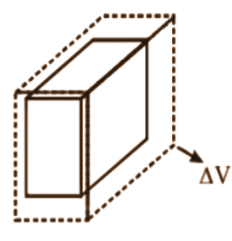$\dfrac{\text{ }\!\!\Delta\!\!\text{ V}}{\text{V}}=\text{ }\!\!\gamma\!\!\text{ }\Delta \text{T}$ Coefficient of volume expansion: It rises in volume per unit volume per degree increase in temperature.

Units of $\text{ }\!\!\alpha\!\!\text{ , }\!\!\beta\!\!\text{ , }\!\!\gamma\!\!\text{ }=\text{ }/\text{ }\!\!{}^\circ\!\!\text{ C or }/\text{K}$

In general, as the volume changes, the density changes as well.

• $\text{ }\!\!\alpha\!\!\text{ }$ is generally higher for metals than $\text{ }\!\!\alpha\!\!\text{ }$ for nonmetals

• $\text{ }\!\!\gamma\!\!\text{ }$ is nearly constant at high temperatures but varies with temperature at low temperatures.

### 1.3 Thermal Expansion:

Most materials, it has been observed, expand when heated and contract when cooled. This expansion is multidimensional.

It has been demonstrated experimentally that a fractional change in any dimension is proportional to a change in temperature.

### Coefficient of Volume Expansion of Cu as a Function of Temperature:

• For ideal gases, $\gamma$ is inversely related to the temperature at constant pressure.

$\text{V = }\dfrac{\text{nRT}}{\text{P}}$

$\Rightarrow \dfrac{\Delta \text{V}}{\text{V}}\text{ = }\dfrac{\Delta \text{T}}{\text{T}}$

$\Rightarrow \text{ }\!\!\gamma\!\!\text{ = }\dfrac{\bot }{\text{T}}$

Water, on the other hand, contracts when heated from $\text{0 }\!\!{}^\circ\!\!\text{ C to 4 }\!\!{}^\circ\!\!\text{ C}$ and thus its density rises from $\text{0 }\!\!{}^\circ\!\!\text{ C to 4 }\!\!{}^\circ\!\!\text{ C}$. This is known as anomalous expansion.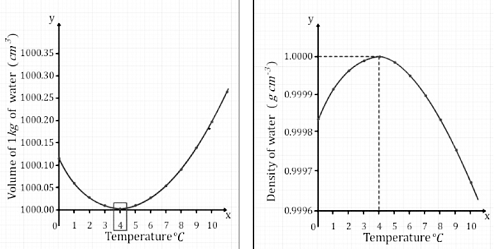• In general $\text{ }\!\!\gamma\!\!\text{ }=\text{ }3\text{ }\!\!\alpha\!\!\text{ = }\dfrac{\text{3}}{2}\text{ }\!\!\beta\!\!\text{ }$

Proof: Consider a cube of length l that expands equally in all directions when its temperature rises by a small $\text{ }\!\!\Delta\!\!\text{ T;}$

We have

$\Delta l\text{ }=\text{ }\!\!\alpha\!\!\text{ }l\Delta \text{T}$

Also,

$\Delta \text{V}=\text{ }{{(l\Delta l)}^{3}}\text{ }\text{ }{{l}^{3}}\text{ }$

$=\text{ }{{l}^{3}}\text{ }+\text{ }3{{l}^{2}}\Delta l\text{ }+\text{ }3l\Delta {{l}^{2}}\text{ }+\text{ }\Delta {{l}^{2}}\text{ }\text{ }{{l}^{3}}\text{ }$

$=\text{ }3{{l}^{2}}\Delta l\text{ }...\left( 1 \right)$

In Equation (1) we ignore $3l\Delta {{l}^{2}}\text{ }\And \Delta {{l}^{3}}\text{ as }\Delta l$ is very small as compared to l.

So,

$\Delta \text{V = }\dfrac{3\text{V}}{l}\Delta l$

$=3\text{V }\!\!\alpha\!\!\text{ }\!\!\Delta\!\!\text{ T }\left[ \text{Using }\dfrac{\text{V}}{l}=\text{ }{{l}^{\text{2}}} \right]\cdots \cdots \left( 2 \right)$

$\dfrac{\Delta \text{V}}{\text{V}}\text{ = 3 }\!\!\alpha\!\!\text{ }\!\!\Delta\!\!\text{ T}$

Similarly, we can prove for area expansion coefficient of thermal expansion is prevented inside the rod by rigidly fixing its ends, then the rod acquires a compressive strain due to external fones at the ends. The corresponding stress set up in the rod is called thermal stress.

Hence, thermal stress $=\frac{F}{A}=Y\left(L-L_{0}\right) / L_{0}$ where Y is Young's modulus of the given material.

This can be simplified into $Y(\alpha \Delta T) / L_{0}$.

Practical applications include railway tracks, metal tyres on cart wheels, bridges, and a variety of other structures.

### 1.4 Heat and Calorimetry:

When two systems at different temperatures are linked together, heat flows from the higher temperature to the lower temperature until their temperatures become the same.

The principle of calorimetry states that heat lost by a body at a higher temperature equals heat gained by a body at a lower temperature, ignoring heat loss to surroundings.

When heat is applied to anybody, either its temperature or its state changes.

#### 1.4.1 Change in Temperature:

On heating, when the temp changes, then

$\text{Heat supplied }\propto \text{ change in temp (}\Delta \text{T)}$

$\text{ }\propto \text{amount of substance }\left( \text{m / n} \right)$

$\text{ }\propto \text{nature of substance }\left( \text{s / C} \right)$

$\text{ }\!\!\Delta\!\!\text{ H = ms }\!\!\Delta\!\!\text{ T}$

Here, $\text{m}$ is the mass of body, $\text{s}$ is specific heat capacity per kg, $\text{ }\!\!\Delta\!\!\text{ T}$ is change in temp

or $\text{ }\!\!\Delta\!\!\text{ H = nC }\!\!\Delta\!\!\text{ T}$

Here, n is the number of moles, C is the Specific/Molar heat Capacity per mole, $\text{ }\!\!\Delta\!\!\text{ T}$ is the change in temp.

#### Specific Heat Capacity:

• The amount of heat needed to increase the temperature of a substance's unit mass by one degree.

• Units:

i. $\text{SI }\to \text{ J / KgK }{{\text{S}}_{{{\text{H}}_{\text{2}}}\text{O}\left( \text{e} \right)}}\text{ = 1 cal / g}{}^\circ \text{C}$

ii. $\text{Common }\to \text{ Cal / g}{}^\circ \text{C }{{\text{S}}_{{{\text{H}}_{\text{2}}}\text{O}\left( \text{ice} \right)}}\text{ = 0}\text{.5 cal / g}{}^\circ \text{C}$

### Molar Heat Capacity:

• The amount of heat required to raise the temperature of a unit mole of a substance by one degree.

• Units:

i. $\text{SI }\to \text{ J / mol K}$

ii. $\text{Common }\to \text{ Cal / gc }\!\!{}^\circ\!\!\text{ }$

### Heat Capacity:

• The amount of heat needed to raise a system's temperature by one degree.

$\Rightarrow \Delta \text{H = S}\Delta \text{T}$ where S is heat capacity. Units

$\text{SI }\to \text{ J / K }$

$\text{Common }\to \text{ Cal / C }\!\!{}^\circ\!\!\text{ }$

• For ${{\text{H}}_{\text{2}}}\text{O}$, the specific heat capacity varies, but only slightly.

• Materials with a higher specific heat capacity require a large amount of heat for a given temperature.

### 1.4.2 Change in State:

On heating, when the phase changes then the heat supplied is directly related to the amount of substance that changes the state (M) and the nature of the substance (L).

$\Rightarrow \Delta \text{H = mL}$

Where L is the latent Heat of process.

• Latent Heat: The amount of heat required to change the state of any substance per mass.

Units:

$\text{SI }\to \text{ J/Kg}$

$\text{Common }\to \text{ Cal/g}$

• The change in state always occurs at a constant temperature.

For example:

$\text{Solid }\rightleftharpoons \text{ Liq }{{\text{L}}_{\text{f}}}$

$\text{Liq }\rightleftharpoons \text{ Gas }{{\text{L}}_{\text{v}}}$

${{\text{L}}_{\text{f}}}\text{ = Latent Heat of fusion}$

${{\text{L}}_{\text{v}}}\text{ = Latent heat of vaporization}$

• If a material is not at its B.P. or M.P, heating will cause the temperature to change until a specific state change temperature is reached.

For Example:  If water is initially at $50\text{ }\!\!{}^\circ\!\!\text{ C}$ at 1 atm pressure in its solid state, then on heating

Step 1: Make a temperature change to the first to $\text{0 }\!\!{}^\circ\!\!\text{ C}$.

Step 2: The ice melts to ${{\text{H}}_{\text{2}}}\text{O }\left( \text{l} \right)$ while the temperature remains constant.

Step 3: The temperature is inverted to $\text{100 }\!\!{}^\circ\!\!\text{ C}\text{.}$

Step 4: ${{\text{H}}_{\text{2}}}\text{O }\left( \text{l} \right)$ boils to steam while the temperature remains constant.

Step 5: Increase the temperature further.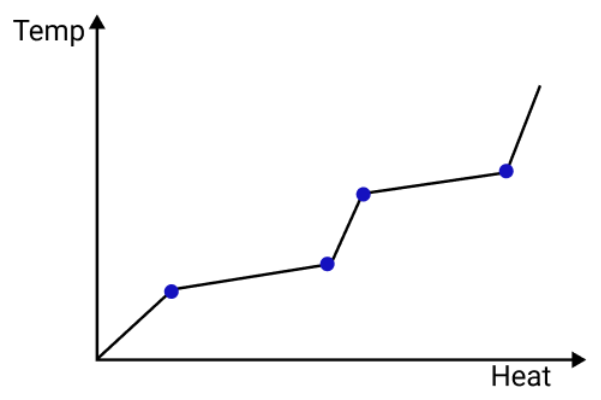• The slope is inversely related to the heat capacity.

• The length of the horizontal line totally depends upon mL for the process.

### 1.4.3 Pressure dependence on melting point and boiling point:

• The melting point decreases with increasing pressure for some substances and increases for others.

• The melting point increases with increasing temperature. The above results can be seen using phaser diagrams.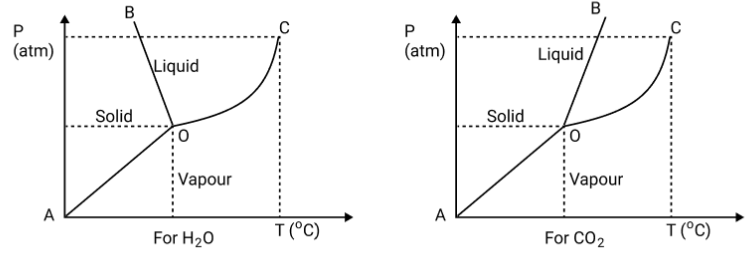Line AO is the sublimation curve, line OB is the fusion curve, line OC is the vapourisation curve, point O is triple point, point C is critical temperature

Triple Point:

The pressure and temperature combination at which all three states of matter (solids, liquids, and gases) coexist. Its value is 273.16 K and 0.006 atm for ${{\text{H}}_{\text{2}}}\text{O}\text{.}$

The combination of pressure and temperature beyond which vapour cannot be liquified is referred to as the critical point. The corresponding temperature and pressure are referred to as the critical temperature and critical pressure.

• The phasor diagram shows that the melting point of H2O decreases as pressure increases. The concept of regulation is based on this.

• Regulation: The phenomenon of refreezing water that has melted below its normal melting point due to the addition of pressure. Cooking on mountains is difficult due to the pressure effect on melting point, whereas cooking in a pressure cooker is easier.

### 1.5 Heat Transfer:

There are three modes of heat transfer.

• Conduction

• Convection

### 1.5.1 Conduction

Thermal conduction is the process by which thermal energy is transferred from the hotter to the colder part of a body or from a hot body to a cold body in contact with it without the transfer of material particles.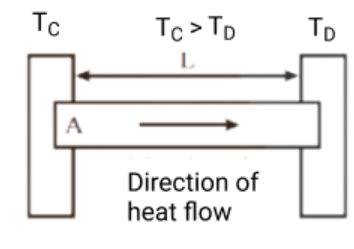The rate of heat energy flowing through the rod becomes constant at steady state. It is given by,

$\text{Q = kA}\dfrac{\left( {{\text{T}}_{\text{C }}}\text{- }{{\text{T}}_{\text{D}}} \right)}{\text{L}}$

This is the rate for rods with a uniform cross-section.

Here, Q is the rate of heat energy flow, A is an area of cross-section, ${{\text{T}}_{\text{C}}}\text{ and }{{\text{T}}_{\text{D}}}$ are the temperature of the hot end and cold end respectively, L is the length of the rod, K is coefficient of thermal conductivity.

Some of the examples of conduction that we experience in our day to day life are being burnt after touching a stove. Your hand is being cooled with ice. By putting a red-hot piece of iron into the water, it is brought to a boil.

### Coefficient of Thermal Conductivity:

• It is defined as the amount of heat conducted in unit time during a steady-state through a unit area of any cross-section of a substance under a unit temperature gradient, with the heat flow being normal to the area.

• Units:

$\text{SI }\to \text{ J / mSk or W/mK}$

• The greater the thermal conductivity, the faster heat energy flows for a given temperature difference.

• Metals have higher thermal conductivity than nonmetals.

• Insulators have a very low thermal conductivity. As a result, heat energy cannot be easily conducted through air.

• The concept of equivalent thermal conductivity of the composite rod can be used for combinations of rods between two ends kept at different temperatures.

${{\text{K}}_{\text{eq}}}$ is the equivalent thermal conductivity of the composite.

• The term $\dfrac{\left( {{\text{T}}_{\text{C }}}\text{- }{{\text{T}}_{\text{D}}} \right)}{\text{L}}$ is known as temperature Gradient.

• Temperature Gradient refers to the decrease in temperature per unit length in the direction of heat energy flow.

• Units:

$\text{SI }\to \text{ K/m}$

• The term Q is the rate of flow of heat energy can also be named as heat current

• The term $\left( \text{L / KA} \right)$  is known as thermal resistance of any conducting rod.

### Thermal Resistance:

• It is defined as the medium's obstruction of the flow of heat current.

• Units:

$\text{SI }\to \text{ K/W}$

### 1.5.2 Convection:

Thermal convection is the process by which heat is transferred from one point to another by the actual movement of heated material particles from a higher-temperature location to a lower-temperature location.

• Forced convection occurs when the medium is forced to move by means of a fan or a pump. Natural or free convection occurs when material moves due to differences in density in the medium.

• Examples of forced convection are the circulatory system, cooling system, and heat connector of an automobile

• Examples of natural convection are trade winds, sea breeze/land breeze, monsoons, and tea burning.

It is a method of heat transmission in which heat travels directly from one location to another without the use of an intermediary medium.

• This radiation of heat energy takes the form of EM waves.

• These radiators are emitted as a result of their temperature, similar to how a red-hot iron or a filament lamp emits light.

• Everybody both radiates and absorbs energy from their surroundings. The amount of energy absorbed is proportional to the colour of the body.

• Black-body radiation is the thermal electromagnetic radiation emitted by a black body within or surrounding a body in thermodynamic equilibrium with its environment (an idealized opaque, non-reflective body). It has a specific spectrum of wavelengths that are inversely related to intensity and are only affected by the body's temperature, which is assumed to be uniform and constant for the sake of calculations and theory.

Stefan's Law states that the radiated power density of a black body is directly related to its absolute temperature T raised to the fourth power.

### Newton's Law of Cooling:

According to Newton’s Law of cooling, the rate of loss of heat, that is, $-\dfrac{\text{d}}{\text{dt}}$ of the body is directly related to the temp difference.

Now,$\text{- }\dfrac{\text{ds}}{\text{dt}}\text{= k }\left( {{\text{T}}_{\text{2}}}\text{ - }{{\text{T}}_{\text{1}}} \right)\text{ }\cdots \cdots \left( 4 \right)$

Here, k is a positive constant which depends on the area and nature of the surface of the body.

Suppose a body of mass m, specific heat capacity s is at temperature ${{\text{T}}_{\text{2}}}\text{ }\!\!\And\!\!\text{ }{{\text{T}}_{\text{1}}}$ be the temperature of surroundings if $\text{d}{{\text{T}}_{\text{2}}}$ the fall of temperature in time dt.

The amount of heat lost is given by,

$\text{dcs = msd}{{\text{T}}_{\text{2}}}$

Therefore, the rate of loss of heat is given by

$\dfrac{\text{dcs}}{\text{dt}}\text{= ms }\dfrac{\text{d}{{\text{T}}_{\text{2}}}}{\text{dt}}\text{ }\cdots \cdots \left( 5 \right)$

From Equation 4 and 5

$-\text{ms}\dfrac{\text{d}{{\text{T}}_{\text{2}}}}{\text{dt}}\text{= k }\left( {{\text{T}}_{\text{2}}}-{{\text{T}}_{\text{1}}} \right)$

$\dfrac{\text{d}{{\text{T}}_{\text{2}}}}{\left( {{\text{T}}_{\text{2}}}-{{\text{T}}_{\text{1}}} \right)}\text{ = -}\dfrac{\text{k}}{\text{ms}}\text{ dt }$

$\text{ = -Kdt }$

Here, $\text{K}=\dfrac{\text{k}}{\text{ms}}$

On integrating,

$\log \left( {{\text{T}}_{\text{2}}}-{{\text{T}}_{\text{1}}} \right)\text{ = -Kt + }\text{C}$

${{\text{T}}_{\text{2}}}\text{ = }{{\text{T}}_{\text{1}}}\text{ + }{{\text{C}}_{\text{1}}}{{\text{e}}^{-\text{kt}}}\text{ }\cdots \cdots \left( 6 \right)$

The equation (6) allows us to calculate the time it takes a body to cool through a given temperature range.

For small temperature differences, the rate of cooling due to conduction, convection, and radiation combined is proportional to the temperature difference.

### Approximation:

If a body cools from ${{\text{T}}_{\text{a}}}\text{ to }{{\text{T}}_{\text{b}}}$ in t times in a medium with a temperature of ${{\text{T}}_{\text{0}}}\text{,}$then

$\left( {{\text{T}}_{\text{a}}}-{{\text{T}}_{\text{b}}} \right)\text{ = K }\left( \dfrac{{{\text{T}}_{\text{a}}}+{{\text{T}}_{\text{b}}}}{2}-{{\text{T}}_{\text{0}}} \right)$

Newton's law of cooling can be studied experimentally.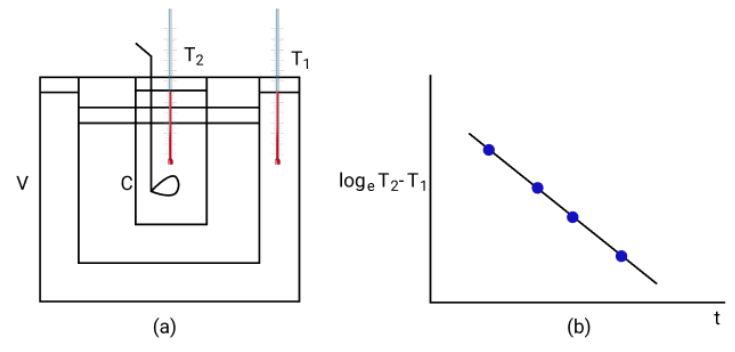### Configuration:

• A double-walled vessel (v) with water contained between two walls.

• Inside the double-walled vessel is a copper calorimeter (c) containing hot water.

• Two thermometers threaded through the carbs are used to measure the temperature ${{\text{T}}_{\text{2}}}$of ${{\text{H}}_{\text{2}}}\text{O}$ in the calorimeter T of water in between the double walls.

### Experiment:

• The temperature of hot water in the calorimeter at equal time intervals.

• As a result, A line graph is drawn between log $\left( {{\text{T}}_{2}}\text{ }{{\text{T}}_{1}} \right)$ and time (t).

• The graph is observed to be a straight line, as predicted by Newton's law of cooling.

## FAQs on Thermal Properties of Matter Class 11 Notes CBSE Physics Chapter 11 (Free PDF Download)

1. What Do You Mean by the Thermal Properties of Matter?

• Thermal properties are the physical properties of matter, which are related to the conductivity of heat.

• Thermal properties explain the response of a material, i.e., how it reacts when subjected to varying heat, either excessive heat or low heat.

2. What is Heat Capacity?

We define heat capacity as the heat required to raise the temperature of a body by 1℃. The amount of heat is expressed in Joule or Calories, while the temperature is expressed in Kelvin/Celsius. The heat capacity of a material is calculated by using the following formula:

Q = mCΔT

Where,

Q = heat capacity in Joules

m = mass in grams

C = specific heat in J/K

ΔT = Change in temperature in K

3. Write the Major Components of the Thermal Properties of Matter.

The major components of thermal properties of matter are:

• Thermal Expansion

• Thermal Stress

• Thermal Conductivity

• Heat Capacity (Q)

4. How Can I Improve My Numerical Solving Ability in Physics?

Our subject experts suggest the following tips to improve your numerical questions solving ability in Physics.

• Go through NCERT numerical type questions to strengthen your concepts.

• Do not jump to a new topic until you’ve solved all the numerical questions related to it.

• Start from the basic concepts, slowly, and gradually move to the advanced topics.

• Keep on practicing until you gain confidence over your numerical solving ability.

5. Give the definition of the following:

• Thermal Conduction

• Coefficient of Thermal Conductivity

• Thermal Conduction –The process of transferring the heat energy from one part of the solid to another part without the actual motion of the particles at a lower temperature is known as thermal conduction or conduction of heat.

• Coefficient of Thermal Conductivity –The amount of heat flowing across the opposite faces of the unit cubes per second is known as the coefficient of the thermal conductivity of a material. This flowing of heat takes place when the opposite faces of the unit cubes are maintained at a temperature difference of 1K.

6. Explain why food in a hot case remains warm for a long time during winters.

The hot vessel is a double-walled vessel. There is an evacuation in the space between the walls of the hot case. The steel is used in making the food container. So, neither the heat from the food container can escape nor the outside air can enter the food container. There is no chance of loss of heat due to radiation because of the highly polished surface of the food container. This is the reason because of which food remains warm in hot cases during winters.

7. Write the different properties of the thermometric substances.

The characteristics of the thermometric substances are:

• The substances should have low freezing points and high boiling points.

• The property of volatility should not be there in the substance.

• The thermal conductivity of the substance should be good.

• For the sensitivity of the substance, the coefficient of expansion should be high.

• Thermometric substances should be available in a pure state.

• For easy calibration, there should be uniformity in the rate of expansion.

• It should be easily accessible.

8. What do you mean by Newton's Law of Cooling? Also, define the cooling curve.

Newton's Law of Cooling tells that the temperature difference between the surroundings and the body is directly proportional to the rate of heat loss per unit surface area of a body.

Note: The temperature difference should not be too high.

The cooling curve is described as a graph between the time and the temperature of the body. The rate of fall of the temperature is given by the tangent drawn to the curve at any point.

9. Why do we use cotton threads, plastic or rubber coverings on steering wheels or handles of cycles or scooters?

As we know metals are good conductors of heat, we use them in making steering wheels or handles of scooters and cycles.

During summers, the temperature of these handles is high and in winters, these have low temperatures so in both cases, there will be heat transfer between these handles and the body.

So, to avoid this transferring of heat these handles and steering wheels are covered with insulators like rubber or plastic. By this, the person is protected from heat or cold.